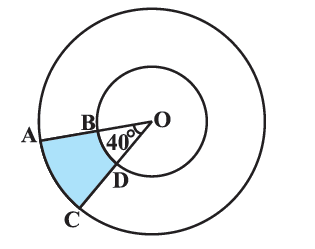In the verge of coronavirus pandemic, we are providing FREE access to our entire Online Curriculum to ensure Learning Doesn't STOP!

# Ex.12.3 Q2 Areas Related to Circles Solution - NCERT Maths Class 10

Go back to  'Ex.12.3'

## Question

Find the area of the shaded region in the given figure, if radii of the two concentric circles with center $$O$$ are $$\text{7 cm}$$ and $$\text{14 cm}$$ respectively and $$\,\angle AOC = {40^ \circ }.$$Video Solution
Areas Related To Circles
Ex 12.3 | Question 2

## Text Solution

What is known?

Radius of $$2$$ concentric circles with centre $$O$$ are $$\text{7 cm}$$ and $$\text{14 cm}$$ and$$\,\angle AOC = {40^ \circ }.$$

What is unknown?

Reasoning:

In a circle with radius r and angle at the center with degree measure $$\theta$$

Area of the sector\begin{align} = \frac{{\rm{\theta }}}{{{{360}^{\rm{o}}}}} \times \pi {r^2}\end{align}

Area of the shaded region can be calculated by subtracting the area of the sector of smaller circle from the area of the sector of the larger circle.

Area of shaded region $$ABDC \,=$$  Area of sector $$ACO\, –$$ Area of sector $$BDO$$

\begin{align}\frac{\theta }{{{{360}^\circ }}} \times \pi {(OA)^2} - \frac{\theta }{{{{360}^\circ }}} \times \pi {(OB)^2}.\end{align}

(Using Area of sector of angle $$\theta = \frac{\theta }{{{{360}^\circ }}} \times \pi {r^2}$$ where r is radius of the circle)

\begin{align}\\&={\frac{{40}}{{{{360}^\circ}}} \times \pi \left( {O{A^2} - {OB^2}} \right)}\\&= {\frac{1}{2} \times \pi {(OA + OB)}(OA - OB)}\\\end{align}

(Using algebraic identity \begin{align}{a^2} - {b^2} = (a + b)(a - b)\end{align} to simplify calculations.)

Steps:

Radius of the larger circle,\begin{align}R = OA = 14cm\end{align}

Radius of the smaller circle,\begin{align}r = OB = 7cm\end{align}

Angle at the center,\begin{align}{\rm{\theta }} = {40^{\rm{\circ}}}\end{align}

Area of shaded region $$ABDC =$$  Area of sector $$ACO\; –$$Area of sector $$BDO$$

\begin{align} &= \frac{\theta }{{{{360}^\circ }}}\!\times\! \pi {(OA)^2} \!-\! \frac{\theta }{{{{360}^\circ }}} \!\times\! \pi {(OB)^2}\\& = \frac{{40}}{{{{360}^\circ }}} \times \pi \left( {{{14}^2} - {7^2}} \right)\\ &= \frac{1}{9} \times \frac{{22}}{7} \times (14 + 7)(14 - 7)\\ &= \frac{{22 \times 21 \times 7}}{{9 \times 7}}\,\,{\text{c}}{{\text{m}}^2}\\& = \frac{{22 \times 7}}{3}\\ &= \frac{{154}}{3}\,{\text{c}}{{\text{m}}^2}\end{align}

Learn from the best math teachers and top your exams

• Live one on one classroom and doubt clearing
• Practice worksheets in and after class for conceptual clarity
• Personalized curriculum to keep up with school